Checkout JEE MAINS 2022 Question Paper Analysis : Checkout JEE MAINS 2022 Question Paper Analysis :

# Lines and Angles Class 9

## CBSE Class 9 Maths Lines and Angles Notes:-

Lines and Angles for class 9 notes are provided here. The chapter lines and angles for class 9 demonstrates the two important shapes in Geometry. As we know, in geometry, we learn about different shapes and sizes. But most of these shapes are formed by the combination of lines and angles. A line is a set of points that has length but no width whereas an angle is formed by joining two rays at a common point called vertex. There are different type of lines and angles introduced in geometry which we are going to learn here. The complete notes on lines and angles are given, which covers the various concepts such as parallel lines, transversal, angles, intersecting lines, interior angles are explained with the examples. Go through the below article to learn about lines and angles.

Also read: Lines and Angles Introduction

## Basic Terms and Definitions on Lines and Angles

Line Segment: A line that has two endpoints is called line-segment.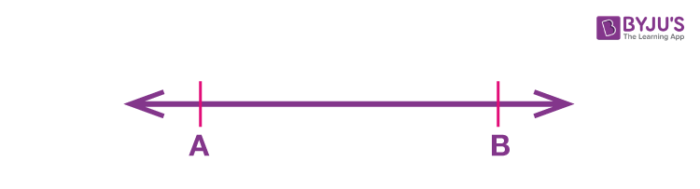Ray: A line with one end point and other end of the line extends up to infinity, then it is called a ray.Collinear points: When three or more points lie on the same line, then they are said to be collinear

Non collinear points: When three or more points does not lie on the same line, then they are non-collinear

Angle: An angle is formed by two rays meeting at a common point (called vertex) and the rays forming angle called arms of tha angle

Acute Angle: An angle that measures between 0° and 90° is called acute angle

Obtuse angle: An angle that measures between 90° and 180° is called obtuse angle

Right angle: An angle that equal to 90° is called right angle

Reflex angle: An angle greater than 180° but less than 360° is called reflex angle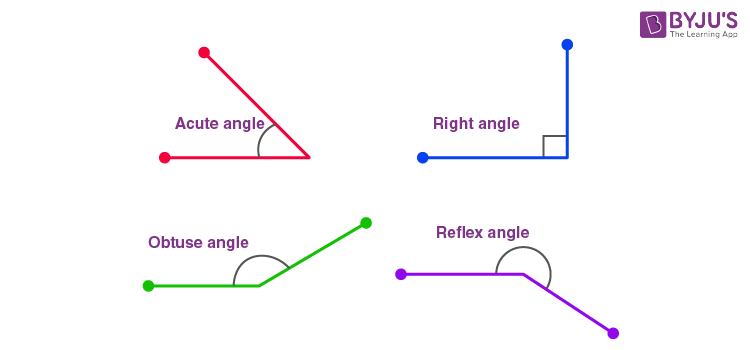Complementary angles: When sum of two angles is equal to 90°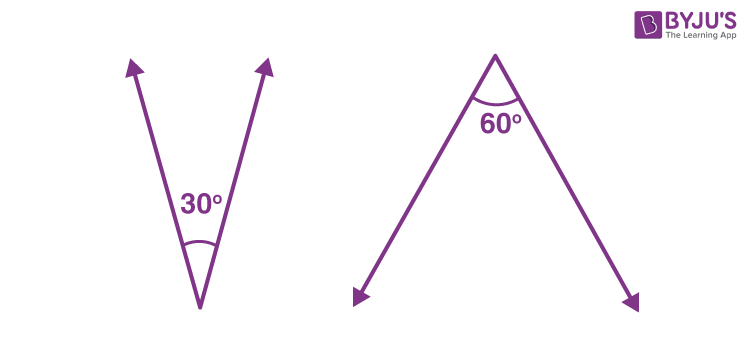Supplementary angles: When sum of two angles is equal to 180°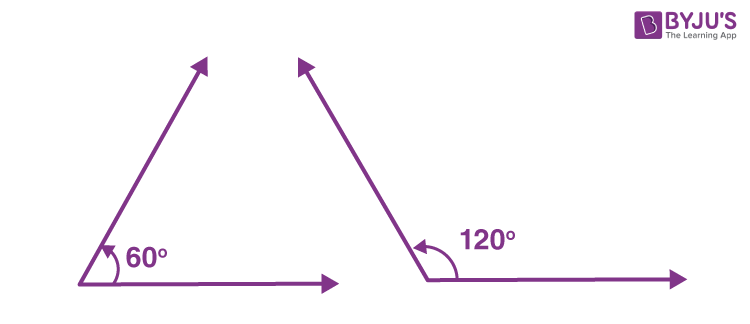Also read: Supplementary And Complementary Angles

Adjacent angles: Two angles with a common vertex, a common arm and their non-common arms are on different sides of the common arm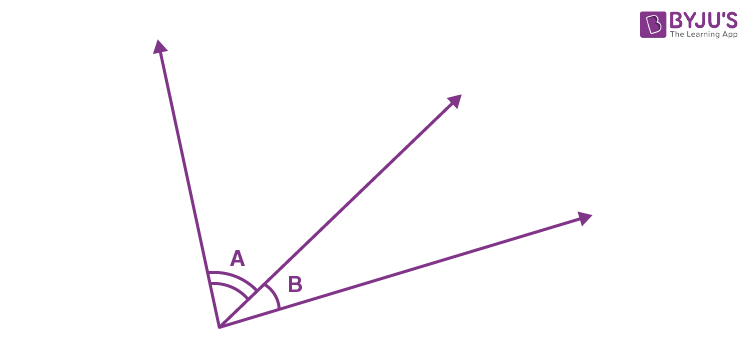Linear pairs of angles: When 2 adjacent angles are supplementary, i.e they form a straight line (add up to 180∘), they are called a linear pair.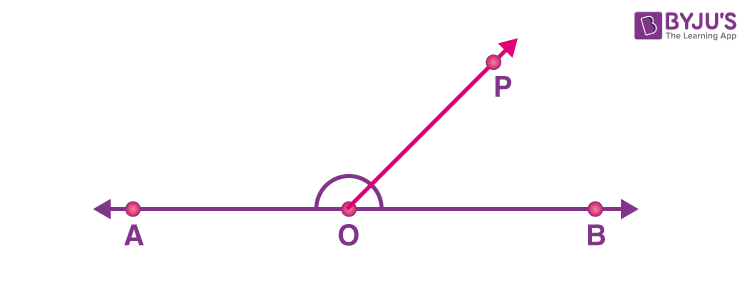Vertically opposite angles: When two lines intersect at a point, they form equal angles that are vertically opposite to each other.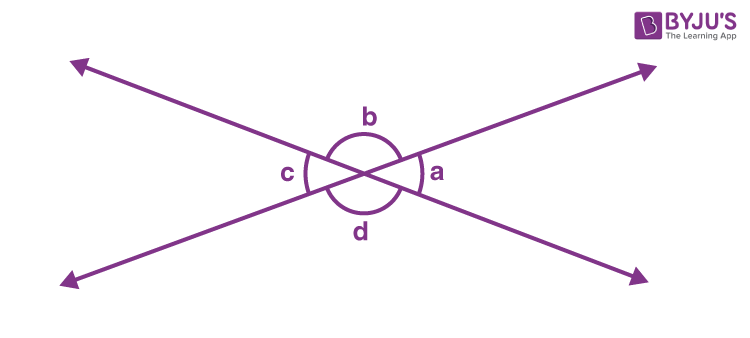Also read: Lines And Angles – Basic Terms

## Intersecting and Non Intersecting Lines

• When two lines intersects each other at a common point then they are said to be intersecting lines.
•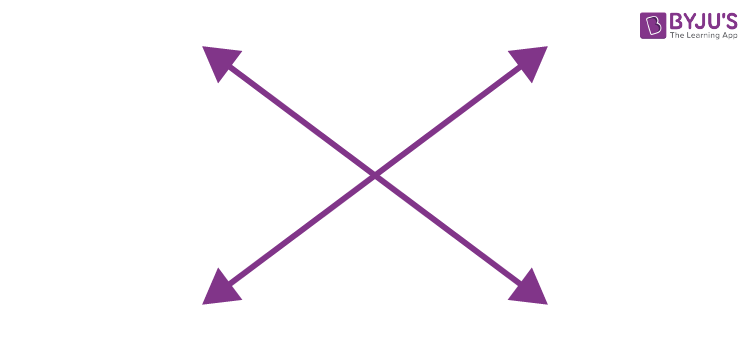• Non intersecting lines are nothing but the parallel lines, that do not intersect each other at a common point.
•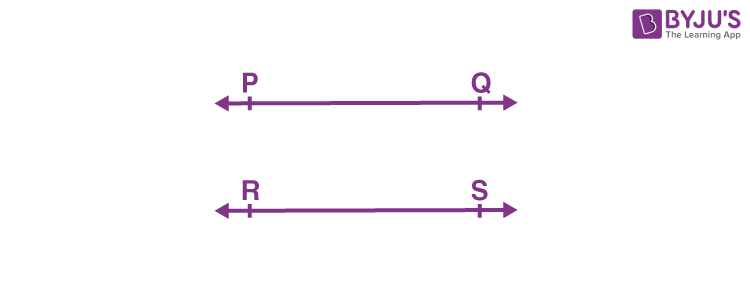#### For More Information On Parallel Lines and Intersecting Lines, Watch The Below Video: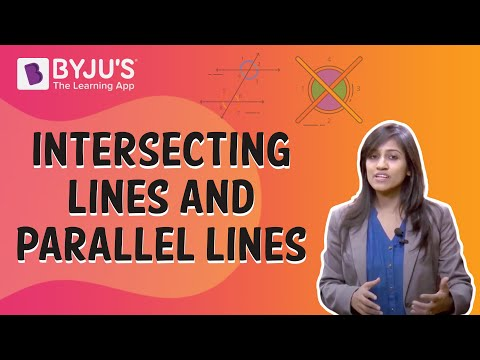## Pairs of Angles

Above we have learned the different type of pairs of angles such as:

• Complementary angles
• Supplementary angles
• Linear pair of angles

Let us discuss the axioms related to all these pairs of angles.

### Axiom- Linear Pair of Angles

If a ray stands on a line, then the sum of two adjacent angles so formed is 180°.

### Axiom- Converse of Linear Pair of Angles

If the sum of two adjacent angles is 180°, then the non-common arms of the angles form a line.

### Theorem – Vertically Opposite Angles

If two lines intersect each other, then the vertically opposite angles are equal.

### Parallel lines with a transversal

A line that intersects two or more lines is called transversal.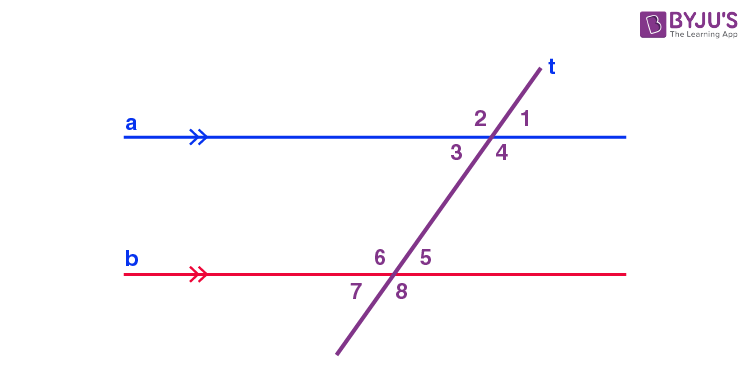• ∠1=∠5,∠2=∠6,∠4=∠8 and ∠3=∠7(Corresponding angles)
• ∠3=∠5,∠4=∠6 (Alternate interior angles)
• ∠1=∠7,∠2=∠8 (Alternate exterior angles)

Interior angles on the same side of the transversal are said to as consecutive interior angles or allied angles or co-interior angles.

### Corresponding angles axiom

If a transversal intersects two parallel lines, then each pair of corresponding angles is equal.

### Converse of corresponding angles axiom

If a transversal intersects two lines such that a pair of corresponding angles is equal, then the two lines are parallel to each other.

### Theorem – Alternate Interior Angles

If a transversal intersects two parallel lines, then each pair of alternate interior angles is equal.

### Theorem – Converse of Alternate Interior Angles

If a transversal intersects two lines such that a pair of alternate interior angles is equal, then the two lines are parallel.

### Theorem – Sum of Co-interior angles is supplementary

If a transversal intersects two parallel lines, then each pair of interior angles on the same side of the transversal is supplementary.

### Theorem – Converse of Sum of Co-interior angles is supplementary

If a transversal intersects two lines such that a pair of interior angles on the same side of the transversal is supplementary, then the two lines are parallel.

## Lines parallel to the same line

Lines that are parallel to the same line are also parallel to each other.

### Theorem – Lines Parallel to the Same Line

Lines which are parallel to the same line are parallel to each other.

## Angle Sum Property of a Triangle

Theorem 1: The sum of the angles of a triangle is 180º.
Theorem 2: If a side of a triangle is produced, then the exterior angle so formed is equal to the sum of the two interior opposite angles.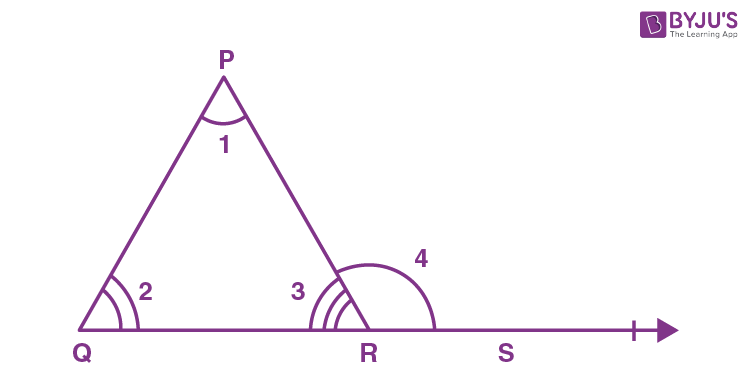∠4=∠1+∠2

#### For More Information On Properties Of Triangle, Watch The Below Video.To know more about Properties of a Triangle, visit here.